# Exercise 18.1 - Solution¶

## Generation of fashion images using Generative Adversarial Networks¶

Let us start to learn how to implement (Generative Adversarial Networks) GAN, as introduced by Goodfellow et al. in Keras, to gain more insights into the training procedure.

As a simple example, we will use GAN to generate small greyscale images. We will use the fashion MNIST data for training the GAN.

1. Pre-train the discriminator and evaluate the test accuracy.
2. Set up the main loop for training the adversarial framework. Use uniform distributed noise as prior distribution for the generator.
3. Generate some images after each training epoch. Plot the training losses for the generator and discriminator and generated images.

Training GANs can be computationally demanding, thus, we recommend to use a GPU for this task.

### Software¶

First we have to import our software.

In :
import numpy as np
import tensorflow as tf
from tensorflow import keras
import matplotlib.pyplot as plt
layers = keras.layers

print("keras version", keras.__version__)

keras version 2.4.0


### Data¶

In :
fashion_mnist = keras.datasets.fashion_mnist

train_images = train_images[...,np.newaxis] / 255.


Let us inspect the data!

In :
class_names = ['T-shirt/top', 'Trouser', 'Pullover', 'Dress', 'Coat',
'Sandal', 'Shirt', 'Sneaker', 'Bag', 'Ankle boot']

plt.figure(figsize=(10,10))

for i in range(16):
plt.subplot(4,4,i+1)
plt.xticks([])
plt.yticks([])
plt.grid(False)
plt.imshow((255 * train_images[i]).astype(np.int).squeeze(), cmap=plt.cm.binary)
plt.xlabel(class_names[train_labels[i]])

plt.show()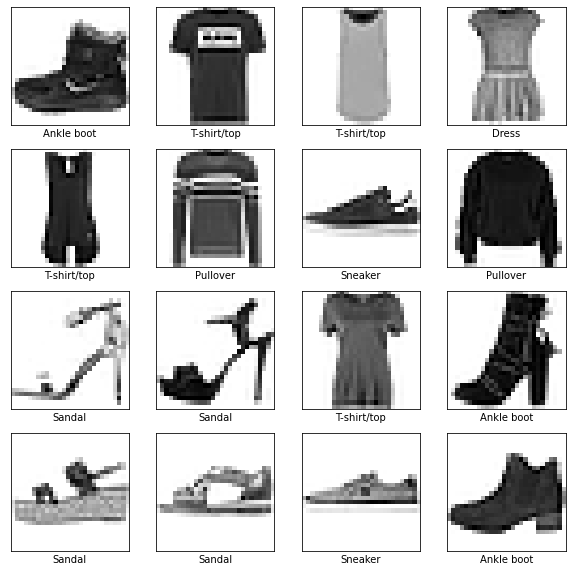### Generator¶

For training GANs we need to further define our generator and discriminator network. We start by defining our generator network, which should map from our noise + label space into the space of images (latent-vector size --> image size). Adding the label the input of to both the generator and discriminator should enforce the generator to produce samples from the according class.

Design a meaningful generator model!
Remember to check the latent and image dimensions. You can make use of the 'DCGAN guidelines'. Use a meaningful final activation function.

In :
def generator_model(latent_size):
""" Generator network """
latent = layers.Input(shape=(latent_size,), name="noise")
z = layers.Dense(7 * 7 * latent_size)(latent)
z = layers.BatchNormalization()(z)
z = layers.Activation('relu')(z)
z = layers.Reshape([7, 7, latent_size])(z)
z = layers.UpSampling2D(size=(2, 2))(z)
z = layers.Conv2D(128, (5, 5), padding='same')(z)
z = layers.BatchNormalization()(z)
z = layers.Activation('relu')(z)
z = layers.UpSampling2D(size=(2, 2))(z)
z = layers.Conv2D(64, (5, 5), padding='same')(z)
z = layers.BatchNormalization()(z)
z = layers.Activation('relu')(z)
z = layers.Conv2D(1, (5, 5), padding='same')(z)
z = layers.Activation('sigmoid')(z)
# We need a sigmoid activation as we normalized the data to [0,1]
return keras.models.Model(latent, z, name="generator")


Build and check the shapes of our generator!

In :
latent_size = 100
g = generator_model(latent_size)
g.summary()

Model: "generator"
_________________________________________________________________
Layer (type)                 Output Shape              Param #
=================================================================
noise (InputLayer)           [(None, 100)]             0
_________________________________________________________________
dense (Dense)                (None, 4900)              494900
_________________________________________________________________
batch_normalization (BatchNo (None, 4900)              19600
_________________________________________________________________
activation (Activation)      (None, 4900)              0
_________________________________________________________________
reshape (Reshape)            (None, 7, 7, 100)         0
_________________________________________________________________
up_sampling2d (UpSampling2D) (None, 14, 14, 100)       0
_________________________________________________________________
conv2d (Conv2D)              (None, 14, 14, 128)       320128
_________________________________________________________________
batch_normalization_1 (Batch (None, 14, 14, 128)       512
_________________________________________________________________
activation_1 (Activation)    (None, 14, 14, 128)       0
_________________________________________________________________
up_sampling2d_1 (UpSampling2 (None, 28, 28, 128)       0
_________________________________________________________________
conv2d_1 (Conv2D)            (None, 28, 28, 64)        204864
_________________________________________________________________
batch_normalization_2 (Batch (None, 28, 28, 64)        256
_________________________________________________________________
activation_2 (Activation)    (None, 28, 28, 64)        0
_________________________________________________________________
conv2d_2 (Conv2D)            (None, 28, 28, 1)         1601
_________________________________________________________________
activation_3 (Activation)    (None, 28, 28, 1)         0
=================================================================
Total params: 1,041,861
Trainable params: 1,031,677
Non-trainable params: 10,184
_________________________________________________________________


We can further plot the model.

In :
keras.utils.plot_model(g, show_shapes=True)

Out: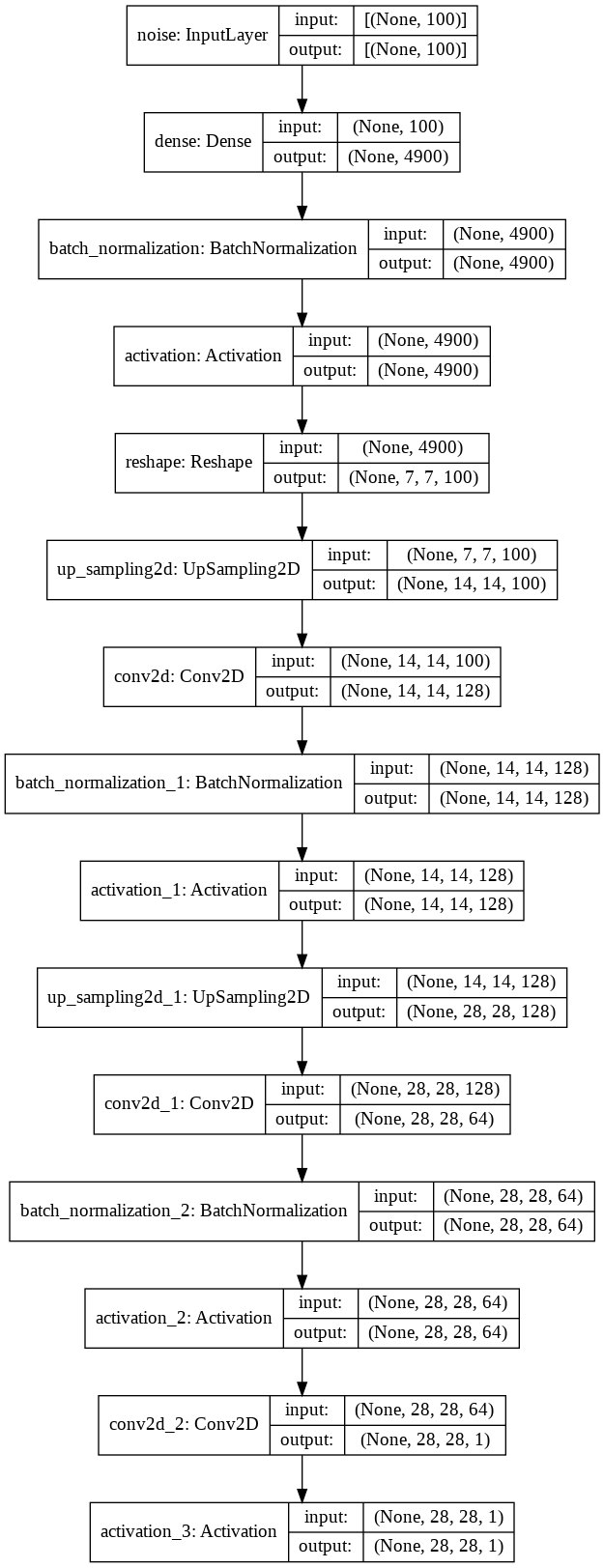### Discriminator¶

The task of the discriminator is to measure the similarity between the fake images (output of the generator) and the real images. So, the network maps from the image space into a 1D space where we can measure the 'distance' between the distributions of the real and generated images (image size --> scalar). Also, here we add the class label to the discriminator.

Design a power- and meaningful critic model!
Remember that you can make use of the DCGAN guidelines (use convolutions!) and check the image dimensions (compare Sec. 18.2.3). We need a softmax as last activation function in the discriminator!

In :
def discriminator_model(drop_rate=0.25):
""" Discriminator network """
image = layers.Input(shape=(28,28,1), name="images")
x = layers.Conv2D(32, (5, 5), padding='same', strides=(2, 2))(image)
x = layers.LeakyReLU(0.2)(x)
x = layers.Conv2D(64, (5, 5), padding='same', strides=(2, 2))(x)
x = layers.LeakyReLU(0.2)(x)
x = layers.Conv2D(128, (5, 5), padding='same', strides=(2, 2))(x)
x = layers.LeakyReLU(0.2)(x)
x = layers.Flatten()(x)
x = layers.Dropout(drop_rate)(x)
x = layers.Dense(256)(x)
x = layers.LeakyReLU(0.2)(x)
x = layers.Dropout(drop_rate)(x)
x = layers.Dense(2)(x)
x = layers.Activation("softmax")(x)
return keras.models.Model(image, x, name="discriminator")

In :
d = discriminator_model()
d.summary()
d.compile(loss='binary_crossentropy', optimizer=d_opt, metrics=["acc"])

Model: "discriminator"
_________________________________________________________________
Layer (type)                 Output Shape              Param #
=================================================================
images (InputLayer)          [(None, 28, 28, 1)]       0
_________________________________________________________________
conv2d_3 (Conv2D)            (None, 14, 14, 32)        832
_________________________________________________________________
leaky_re_lu (LeakyReLU)      (None, 14, 14, 32)        0
_________________________________________________________________
conv2d_4 (Conv2D)            (None, 7, 7, 64)          51264
_________________________________________________________________
leaky_re_lu_1 (LeakyReLU)    (None, 7, 7, 64)          0
_________________________________________________________________
conv2d_5 (Conv2D)            (None, 4, 4, 128)         204928
_________________________________________________________________
leaky_re_lu_2 (LeakyReLU)    (None, 4, 4, 128)         0
_________________________________________________________________
flatten (Flatten)            (None, 2048)              0
_________________________________________________________________
dropout (Dropout)            (None, 2048)              0
_________________________________________________________________
dense_1 (Dense)              (None, 256)               524544
_________________________________________________________________
leaky_re_lu_3 (LeakyReLU)    (None, 256)               0
_________________________________________________________________
dropout_1 (Dropout)          (None, 256)               0
_________________________________________________________________
dense_2 (Dense)              (None, 2)                 514
_________________________________________________________________
activation_4 (Activation)    (None, 2)                 0
=================================================================
Total params: 782,082
Trainable params: 782,082
Non-trainable params: 0
_________________________________________________________________

/usr/local/lib/python3.7/dist-packages/tensorflow/python/keras/optimizer_v2/optimizer_v2.py:375: UserWarning: The lr argument is deprecated, use learning_rate instead.
"The lr argument is deprecated, use learning_rate instead.")


We can further plot the model.

In :
keras.utils.plot_model(d, show_shapes=True)

Out: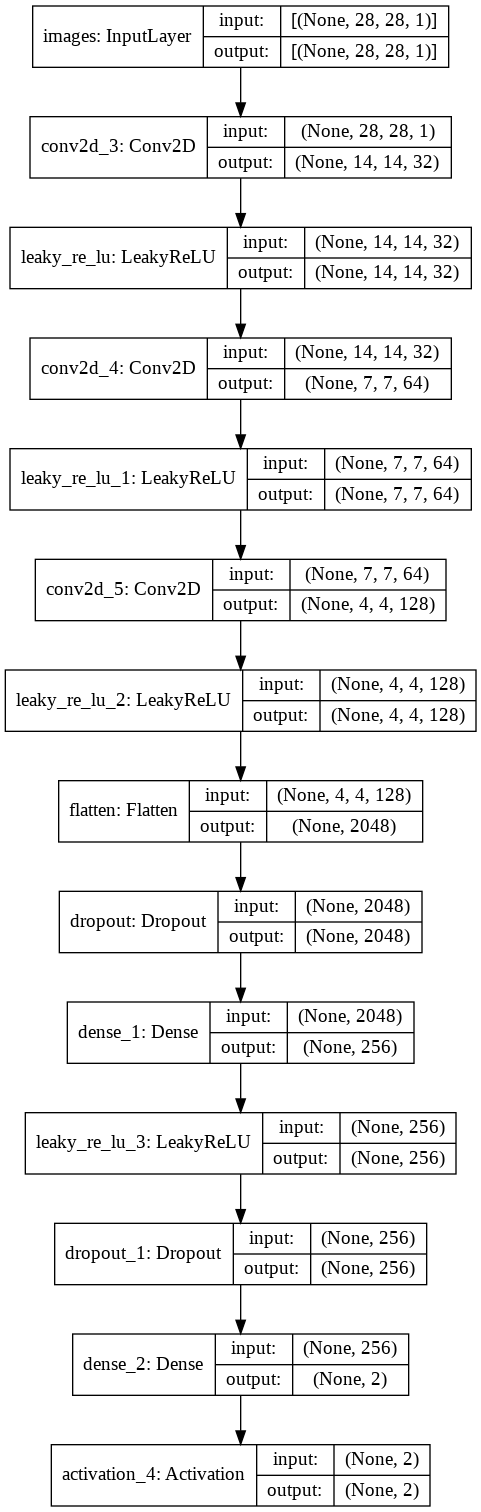### Training preparations¶

After building the generator and discriminator, we have to compile it. But before, we have to freeze the weights of the discriminator. (Remember that we have to fix the discriminator weights for training the generator because we want to fool the discriminator by drawing excellent images, not by making our discriminator a worse classifier).

In :
def make_trainable(model, trainable):
''' Freezes/unfreezes the weights in the given model '''
for layer in model.layers:
# print(type(layer))
if type(layer) is layers.BatchNormalization:
layer.trainable = True
else:
layer.trainable = trainable


Note that after we compiled a model, calling make_trainable will have no effect until compiling the model again.

In :
make_trainable(d, False)  # freeze the critic during the generator training
make_trainable(g, True)  # unfreeze the generator during the generator training


We build the pipeline for the generator training by stacking the generator on the discriminator (with frozen weights).

In :
gen_input = g.inputs
generator_training = keras.models.Model(gen_input, d(g(gen_input)))
generator_training.summary()

Model: "model"
_________________________________________________________________
Layer (type)                 Output Shape              Param #
=================================================================
noise (InputLayer)           [(None, 100)]             0
_________________________________________________________________
generator (Functional)       (None, 28, 28, 1)         1041861
_________________________________________________________________
discriminator (Functional)   (None, 2)                 782082
=================================================================
Total params: 1,823,943
Trainable params: 1,031,677
Non-trainable params: 792,266
_________________________________________________________________

In :
keras.utils.plot_model(generator_training, show_shapes=True)

Out: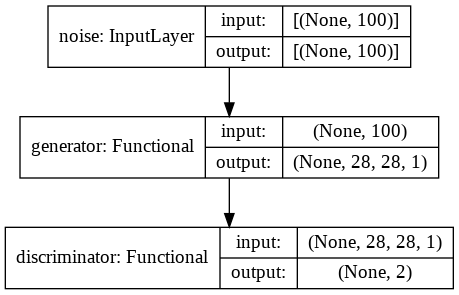In :
g_opt = keras.optimizers.Adam(lr=2e-4, beta_1=0.5, decay=0.0005)
generator_training.compile(loss='binary_crossentropy', optimizer=g_opt)

/usr/local/lib/python3.7/dist-packages/tensorflow/python/keras/optimizer_v2/optimizer_v2.py:375: UserWarning: The lr argument is deprecated, use learning_rate instead.
"The lr argument is deprecated, use learning_rate instead.")


We pre-train the discriminator using 5000 real and 5000 fakes samples (pure noise, since the generator wasn't updated yet).

In :
ntrain = 5000
no = np.random.choice(len(train_images), size=ntrain, replace='False')
real_train = train_images[no,:,:,:]   # sample real images from training set
noise_gen = np.random.uniform(0,1,size=[ntrain, latent_size])
generated_images = g.predict(noise_gen)  # generate fake images with untrained generator

X = np.concatenate((real_train, generated_images))
y = np.zeros([2*ntrain, 2])   # class vector: one-hot encoding
y[:ntrain, 1] = 1             # class 1 for real images
y[ntrain:, 0] = 1             # class 0 for generated images

# - Train the discriminator for 1 epoch on this dataset.
d.fit(X,y, epochs=1, batch_size=64)

157/157 [==============================] - 2s 9ms/step - loss: 0.0666 - acc: 0.9811

Out:
<tensorflow.python.keras.callbacks.History at 0x7ff8001e8d90>

Select a reasonable batch size. Find a good number of epochs.

In :
losses = {"d":[], "g":[]}
discriminator_acc = []
batch_size = 64
nsamples = len(train_images)
iterations_per_epoch = nsamples / batch_size   # Number of training steps per epoch len(train_images)
epochs = 15

iters = 0

for epoch in range(epochs):
print("Epoch: {0:2d}/{1:2d}".format(epoch, epochs))
perm = np.random.choice(nsamples, size=nsamples, replace='False')

for i in range(int(iterations_per_epoch)):

# Create a mini-batch of data (X: real images + fake images, y: corresponding class vectors)
image_batch = train_images[perm[i*batch_size:(i+1)*batch_size],:,:,:]    # real images
noise_gen = np.random.uniform(0.,1.,size=[batch_size, latent_size])

# Generate images using the generator
generated_images = g.predict(noise_gen)

X = np.concatenate((image_batch, generated_images))
y = np.zeros([2*batch_size,2])  # class vector
y[0:batch_size,1] = 1
y[batch_size:,0] = 1

# Train the discriminator on the mini-batch
d_loss, d_acc  = d.train_on_batch(X,y)
losses["d"].append(d_loss)
discriminator_acc.append(d_acc)

# Create a new mini-batch of data (X_: noise, y_: class vectors pretending that these produce real images)
X_ = np.random.uniform(0.,1.,size=[batch_size, latent_size])
y_ = np.zeros([batch_size,2])
y_[:,1] = 1

# Train the generator part of the GAN on the mini-batch
g_loss = generator_training.train_on_batch(X_, y_)
losses["g"].append(g_loss)

iters +=1

if iters % 1000 == 1:
# Plot some fake images
noise = np.random.uniform(0.,1.,size=[16,latent_size])
generated_images = g.predict(noise)
plt.figure(figsize=(5, 5))

for i in range(4):
plt.subplot(2,2,i+1)
plt.xticks([])
plt.yticks([])
plt.grid(False)
img = plt.imshow((255 * generated_images[i]).astype(np.int).squeeze(), cmap=plt.cm.binary)

plt.suptitle("Iteration %i" %iters)
plt.savefig("./fake_fMNIST_iteration_%.6i.png" % iters)

Epoch:  0/15
Epoch:  1/15
Epoch:  2/15
Epoch:  3/15
Epoch:  4/15
Epoch:  5/15
Epoch:  6/15
Epoch:  7/15
Epoch:  8/15
Epoch:  9/15
Epoch: 10/15
Epoch: 11/15
Epoch: 12/15
Epoch: 13/15
Epoch: 14/15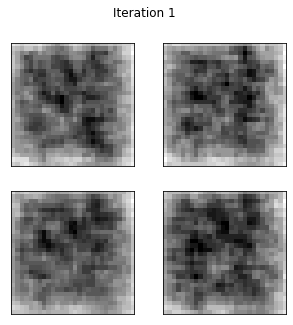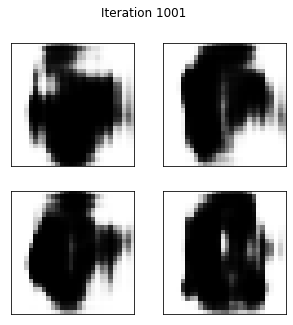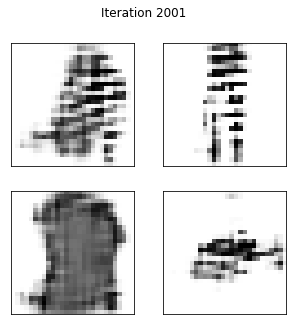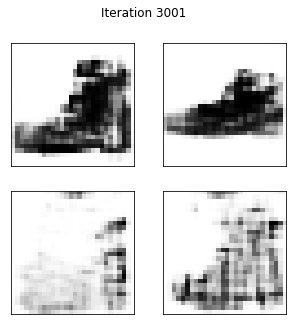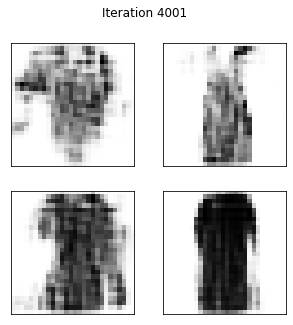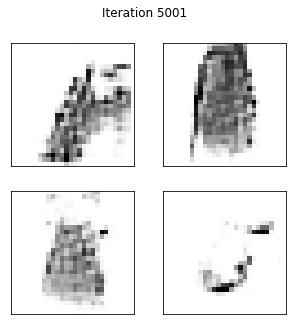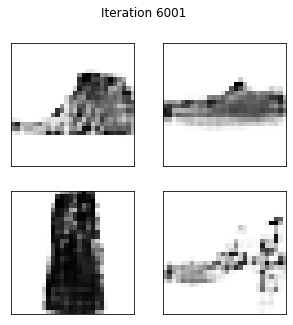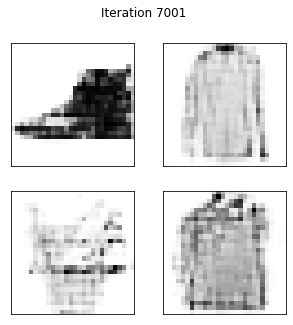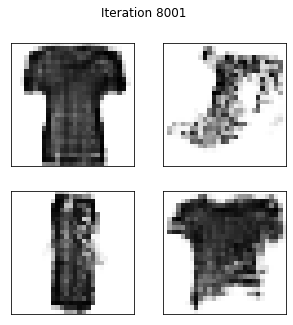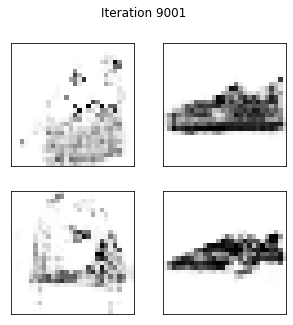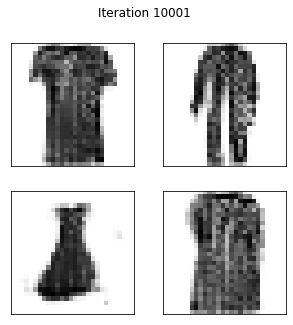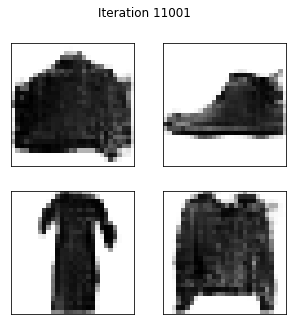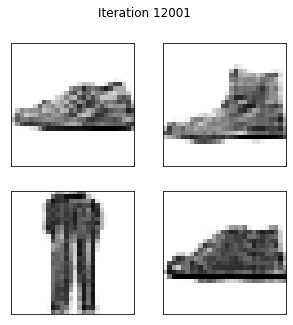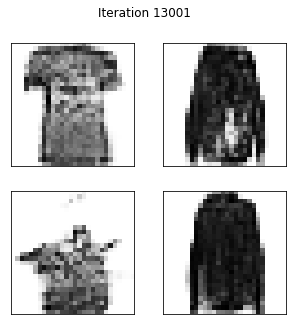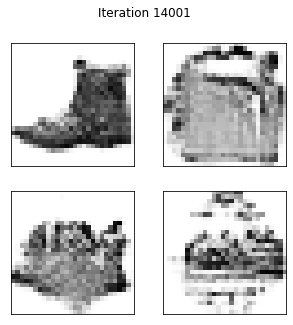After roughly 3,000 iterations, we can recognize the basic shapes of the clothes. Above iteration 10,000, the images are of good quality.

## Plot the training history¶

Plot the loss of the discriminator and the generator as function of iterations.

In :
plt.figure(figsize=(10,8))
plt.semilogy(losses["d"], label='discriminator loss')
plt.semilogy(losses["g"], label='generator loss')
plt.ylabel("loss")
plt.xlabel("iterations")
plt.legend()
plt.show()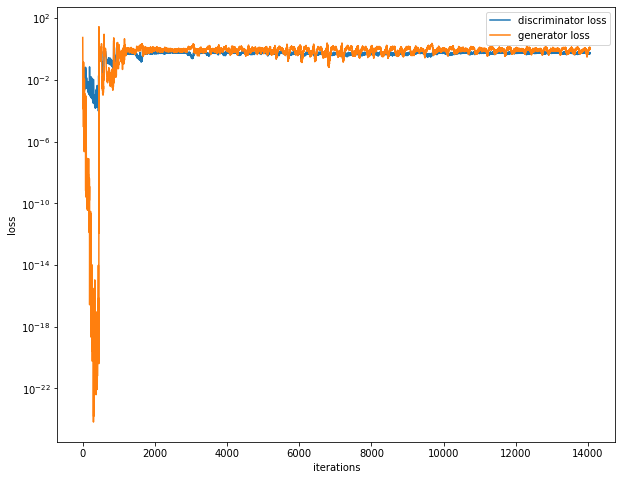Plot the accuracy of the discriminator as function of iterations

In :
plt.figure(figsize=(10,8))
plt.semilogy(discriminator_acc, label='discriminator')
plt.ylabel("accuracy")
plt.xlabel("iterations")
plt.legend()
plt.show()
`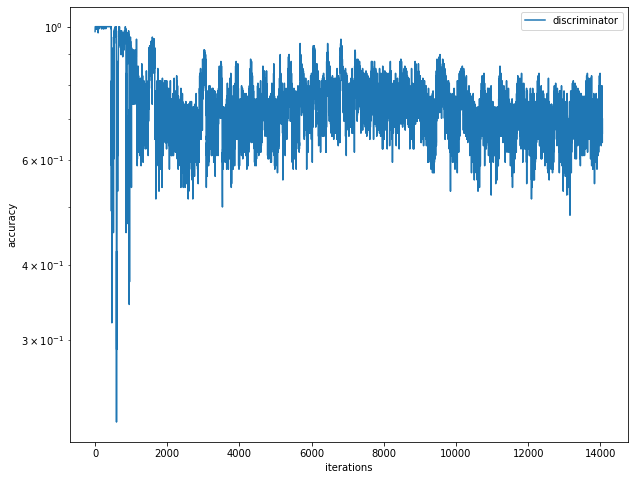Further questions
Check the loss and the generated images.

Does the image quality correlate with the discriminator or the generator loss? Is the generator able to produce all classes of the dataset? How can you improve the performance?|

"The image quality does not correlate with the discriminator or the generator loss. You can further improve the performance using conditioning of the generator and discriminator and adding a decay in the optimizer."